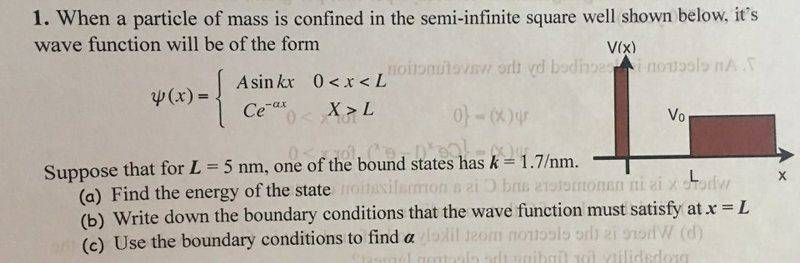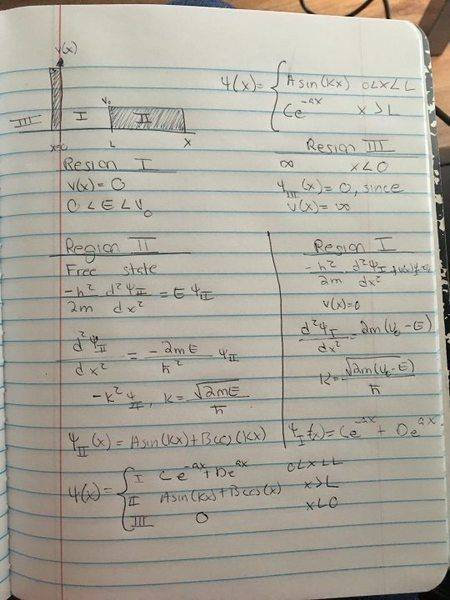# Schrödinger equation

• Ashley1nOnly

## Homework Statement

I have an attachment

## Homework Equations

Schrödinger equation

## The Attempt at a Solution

The issues I am having is how to start this one. This is not a infinite square well but a semi-infinite square well.
I know that energy= K^2= 2mE/h^2
Where h is planks constant 6.626 X 10^-34 J•s
So rearranging (K^2 • h^2)/(2m) =E
How do I find my m and solve the other ones

At X=L
The first derivatives must be equal at X=L
Asin(kL)=Ce^(-aL)
KAcos(kL)=-aCe^(-aL)

Am I going in the right directionI think this belongs in the advanced physics forum.